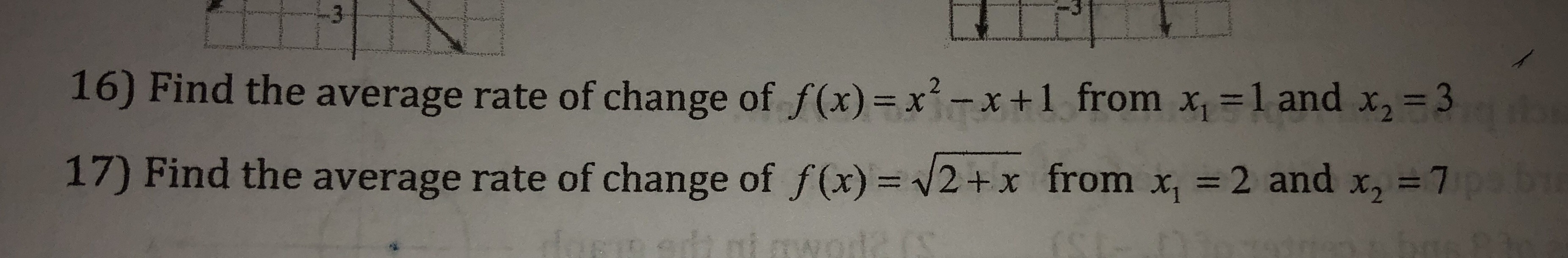16) Find the average rate of change of f(x)xx+1 from x 1 and x,-317) Find the average rate of change of f(x) = +x from x| = 2 and x,-7

Question

for #16help_outlineImage Transcriptionclose16) Find the average rate of change of f(x)xx+1 from x 1 and x,-3 17) Find the average rate of change of f(x) = +x from x| = 2 and x,-7 fullscreen
Step 1

Average rate of change of a function f(x) over an interval [a, b] can be found using the formula (f(b) - f(a))/(b - a)

Step 2

Let us find f(3) and f(1), substitute them in the formula to find the average rate of change of ...

Want to see the full answer?

See Solution

Want to see this answer and more?

Our solutions are written by experts, many with advanced degrees, and available 24/7

See Solution
Tagged in

Algebra